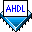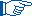## In-Line Logic Function Reference

 See AlsoAn in-line logic function reference is a Boolean equation that implements a logic function. It is a shorthand method for implementing a logic function that uses only one line of the Logic Section and does not require an Instance Declaration in the Variable Section.

When you wish to implement an instance of a megafunction or macrofunction with an in-line logic function reference, you must first ensure that the function's logic is defined in its own design file and a Function Prototype Statement specifies the ports and parameters of the function.

You can also implement an instance of a primitive with an in-line logic function reference. However, in contrast to megafunctions and macrofunctions, primitive logic is predefined, so you do not need to define the primitive logic in a separate design file. In most cases, a Function Prototype Statement is not needed. See Function Prototype Statement for more information.Instance Declarations provide named nodes that are useful for entering resource assignments and simulating a project. In contrast, when an in-line logic function reference does not define an instance name, the instance's node names—which are based on the instance name—may change as the project logic changes. Node names do not change for an in-line logic function reference that defines an instance name.

The following examples show the Function Prototypes for the `compare` and `lpm_add_sub` functions. The `compare` function has input ports `a[3..0]` and `b[3..0]` and output ports `less`, `equal`, and `greater`; the `lpm_add_sub` function has the input ports `dataa[LPM_WIDTH-1..0]`, `datab[LPM_WIDTH-1..0]`, `cin`, and `add_sub`, and output ports `result[LPM_WIDTH-1..0]`, `cout`, and `overflow`.

```FUNCTION compare (a[3..0], b[3..0])
RETURNS (less, equal, greater);

WITH (LPM_WIDTH, LPM_REPRESENTATION)
RETURNS (result[LPM_WIDTH-1..0], cout, overflow);```

The in-line logic function references for the `compare` and `lpm_add_sub` functions appear on the right of the equations below. The instance name of the `compare` function is `compare1`.

```(clockwise, , counterclockwise) = compare1: compare(position[], target[]);

sum[] = lpm_add_sub (.datab[] = b[], .dataa[] = a[])
WITH (LPM_WIDTH = 8)
RETURNS (.result[]);```

The in-line logic function reference for the DFF primitive appears on the right of the equation below. The instance name of the DFF primitive is `dffex`.

`q = dffex: dff (d, clk, clrn, prn)`

The in-line logic function reference for a logic function has the following characteristics:

• The symbolic name and colon (`:`) immediately to the right of the equals symbol (`=`) defines an instance name for a Quartus® II primitive or user-defined megafunction or macrofunction. In the `compare` example shown above, `compare1:` defines the instance name of the `compare` function as `compare1`. Similarly, in the `dff` example shown above, `dffex:` defines the instance name of the DFF primitive as `dffex`.

• The function name on the right of the symbolic name and colon is followed by a signal list enclosed in parentheses `()`, containing symbolic names, decimal numbers, or groups, separated by commas (`,`). These items correspond to the input ports of the function.

When you specify the function name, you can use the name substitution feature to specify a text string for the name that is replaced by a different text string when the design is compiled. Before you can use the name substitution feature, you must turn on the `NAME_SUBSTITUTION `option in the TDF's Options Statement and specify the replacement string for the name in the TDF's Parameters Statement. You can then specify the text string to be replaced in the in-line logic function using the format `@`<text string>.  Example

• In the signal list, port names can be given through positional port association or named port association:

• In the `compare` example shown above, the `a[3..0]` and `b[3..0]` inputs of `compare` are connected to the variables named `position[]` and `target[]`, respectively, through positional port association. When you use positional port association, you can use commas as placeholders for outputs that are not connected to a variable. In `compare`, the `equal` output is not connected to any variable, so an extra comma is needed to hold its place in the group on the left of the equation.

• In the `lpm_add_sub` example shown above, the `.datab[]` and `.dataa[]` inputs of `lpm_add_sub` are connected to the variables `b[]` and `a[]`, respectively, through named port association. Port names are connected to variables with an equals symbol (`=`).Port names must have the format `.` on the right of in-line logic function references that use named port association. Named port association is supported only on the right of an in-line logic function reference. The left of an in-line logic function reference is always connected to variables by positional port association.
• In a parameterized function, the keyword `WITH` and the parameter name list follows the input port list. The list is enclosed in parentheses; parameter names are separated by commas. Only the parameters used by the instance are declared; optional parameter values are separated from parameter names by an equals symbol. In the `lpm_add_sub` example shown above, the `LPM_WIDTH` parameter is assigned a value of `8`. If no parameter values are assigned in the in-line logic function reference, the Compiler searches for them in the parameter value search order.

• On the left of the in-line logic function reference, the outputs of the function are connected to variables. In the `compare` example shown above, the function's `less` and `greater` outputs are connected to the variables `clockwise` and `counterclockwise`, respectively, through positional port association. Similarly, in the `lpm_add_sub` example, the function's `sum[]` outputs are connected through positional port association.

• The values of the variables, which are determined elsewhere in the Logic Section, feed the associated inputs and outputs. In the `compare` example shown above, the values of `position[]` and `target[]` feed the inputs of `compare`. The values of output ports `less` and `greater` feed `clockwise` and `counterclockwise`, respectively. These variables may be used in other operations in the Logic Section.

• The in-line logic function reference can contain an optional `RETURNS` clause, which is based on the `RETURNS` clause in the Function Prototype. The `RETURNS` clause lists the subset of the function's outputs that is used in the instance. In the `lpm_add_sub` example shown above, the `RETURNS` clause does not contain the `cout` and `overflow` outputs of `lpm_add_sub`; therefore, only the `sum[]` output is used on the left of the in-line logic function reference and comma placeholders are not required for `cout` and `overflow`.